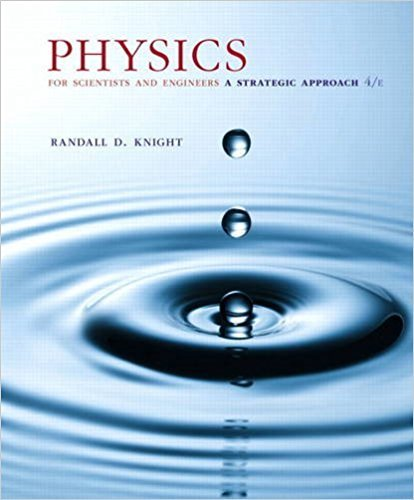×
×

# a. When the displacement of a mass on a spring is 1 2 A,ISBN: 9780134081496 191

## Solution for problem 15.73 Chapter 15

Physics for Scientists and Engineers: A Strategic Approach, Standard Edition (Chs 1-36) | 4th Edition

• Textbook Solutions
• 2901 Step-by-step solutions solved by professors and subject experts
• Get 24/7 help from StudySoup virtual teaching assistantsPhysics for Scientists and Engineers: A Strategic Approach, Standard Edition (Chs 1-36) | 4th Edition

4 5 1 328 Reviews
15
4
Problem 15.73

a. When the displacement of a mass on a spring is 1 2 A, what fraction of the energy is kinetic energy and what fraction is potential energy? b. At what displacement, as a fraction of A, is the energy half kinetic and half potential?

Step-by-Step Solution:
Step 1 of 3
Step 2 of 3

Step 3 of 3

##### ISBN: 9780134081496

Physics for Scientists and Engineers: A Strategic Approach, Standard Edition (Chs 1-36) was written by and is associated to the ISBN: 9780134081496. The answer to “a. When the displacement of a mass on a spring is 1 2 A, what fraction of the energy is kinetic energy and what fraction is potential energy? b. At what displacement, as a fraction of A, is the energy half kinetic and half potential?” is broken down into a number of easy to follow steps, and 45 words. The full step-by-step solution to problem: 15.73 from chapter: 15 was answered by , our top Physics solution expert on 12/28/17, 08:06PM. Since the solution to 15.73 from 15 chapter was answered, more than 230 students have viewed the full step-by-step answer. This textbook survival guide was created for the textbook: Physics for Scientists and Engineers: A Strategic Approach, Standard Edition (Chs 1-36), edition: 4. This full solution covers the following key subjects: . This expansive textbook survival guide covers 42 chapters, and 4463 solutions.

Unlock Textbook Solution